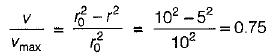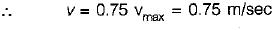Courses

# Laminar Flow In Pipes - 1

## 10 Questions MCQ Test Mock Test Series for Civil Engineering (CE) GATE 2020 | Laminar Flow In Pipes - 1

Description
This mock test of Laminar Flow In Pipes - 1 for Civil Engineering (CE) helps you for every Civil Engineering (CE) entrance exam. This contains 10 Multiple Choice Questions for Civil Engineering (CE) Laminar Flow In Pipes - 1 (mcq) to study with solutions a complete question bank. The solved questions answers in this Laminar Flow In Pipes - 1 quiz give you a good mix of easy questions and tough questions. Civil Engineering (CE) students definitely take this Laminar Flow In Pipes - 1 exercise for a better result in the exam. You can find other Laminar Flow In Pipes - 1 extra questions, long questions & short questions for Civil Engineering (CE) on EduRev as well by searching above.
QUESTION: 1

### In a laminar flow between two static parallel plates, the velocity at mid-point is found to be 2.0 m/s. If the space between the plates is 10 cm, then the discharge per unit width (in m3/s/m) will be

Solution:

The average velocity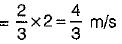The discharge per unit width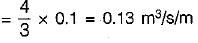QUESTION: 2

### An oil of viscosity 9 poise is flowing through a horizontal pipe of 60 mm diameter. If the flow is laminar, the pressure drop in 100 m length of the pipe is 1800 kN/m2. The average velocity of flow of the oil is

Solution:

1 poise = 0.1 N-s/m2
∴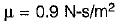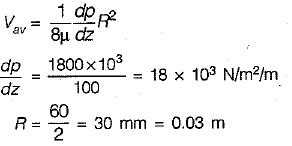∴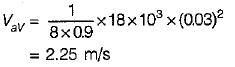QUESTION: 3

### The Chezy's coefficient C is related to Darcy- Weisbach friction factor ‘f’ as

Solution: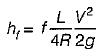(Darcy-Weisbach equation for non- circular section)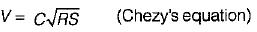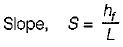Equating both equations, we get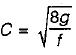QUESTION: 4

A circular pipe of radius R carries a laminar flow of a fluid. The average velocity is indicated as the local velocity at what radial distance, measured from the centre?

Solution:

For laminar flow in a circular pipe, the local velocity may be given as,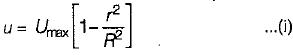For laminar flow in a circular pipe, the average velocity may be given as,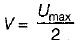......(ii)

Equating (i) and (ii), we get,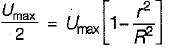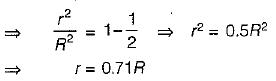QUESTION: 5

The velocity at which the laminar flow ceases, is known as

Solution:

The velocity at which the flow changes from the laminar to turbulent for the case of a given fluid at a given temperature and in a given pipe is known as critical velocity. The state of flow in between these two types of flow is known as ‘transitional state’.

QUESTION: 6

The ratio of maximum velocity to average velocity for steady flow between fixed parallel plates is:

Solution:

For a laminar flow between parallel plates: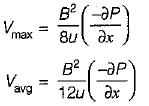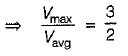QUESTION: 7

The shear stress distribution for a fluid flowing in between the parallel plates, both at rest, is

Solution:

Shear stress distribution between the parallel plates is given by,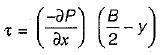It has same maximum value at either boundary and decreases linearly to zero at the mid plane.

QUESTION: 8

The ratio of average velocity to maximum velocity for steady laminar flow in circular pipes is

Solution:
QUESTION: 9

Consider the following statements with reference to steady laminar flow
1. shear stress is zero at the centre.
2. discharge varies directly with viscosity of the fluid flowing.
3. velocity is maximum at the centre.
4. hydraulic gradient varies directly with the velocity.

Which of these statements is/are correct?

Solution: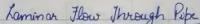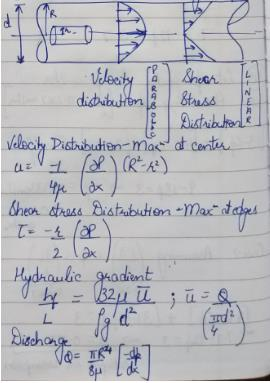QUESTION: 10

In a laminar flow through a circle pipe of diameter 20 cm, the maximum velocity is found to be 1 m/s. The velocity at a radial distance of 5 cm from the axis of the pipe will be

Solution: